Monday, September 27, 2021
Home > Latest Announcement > NMTC 2017 Question Papers With Solutions Primary Level

# NMTC 2017 Question Papers With Solutions Primary Level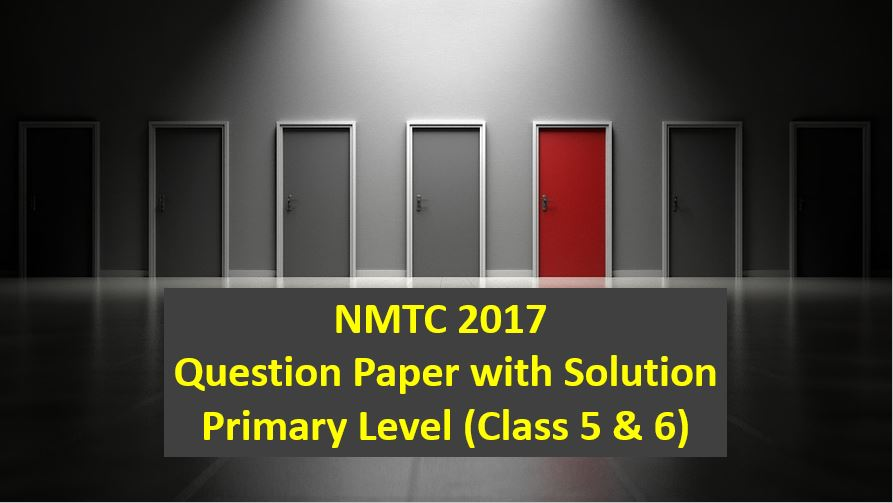# NMTC 2017 Question Papers with Solutions Primary Level

NMTC is an acronym for National Mathematics Talent Contest. NMTC is conducted by AMTI (Association of Mathematics Teachers of India) to identify the talent of the students in Mathematics. In this post, NMTC 2017 Question Papers with Solutions Primary Level is published

Part A: Instruction:

Only One of the choices A, B, C, D is correct. For each correct response, you get 1 mark. For each incorrect response, you lose 1/2 mark.

NMTC 2017 Paper For Primary Level Ques No 1:

Which one of the following numbers in NOT the sum of two prime numbers?

Options:

A. 24

B. 30

C. 67

D. 21

Solution: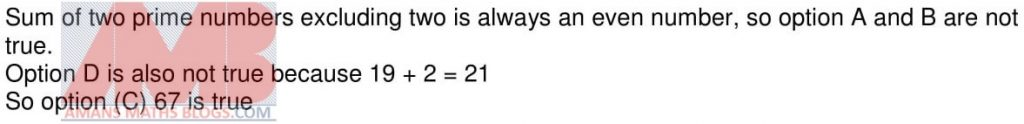NMTC 2017 Paper For Primary Level Ques No 2:

ABCD is square and PB = 2AP. The perimeter of the rectangle APQD is 80 cm. The perimeter of ABCD in cms isOptions:

A. 100

B. 120

C. 140

D. 160

Solution:NMTC 2017 Paper For Primary Level Ques No 3:

Sanket added up all the even numbers from 1 to 101. Then, from the total he obtained, he subtracted all odd numbers between 0 and 100. The answer he would have obtained is

Options:

A. 0

B. 20

C. 30

D. 50

Solution: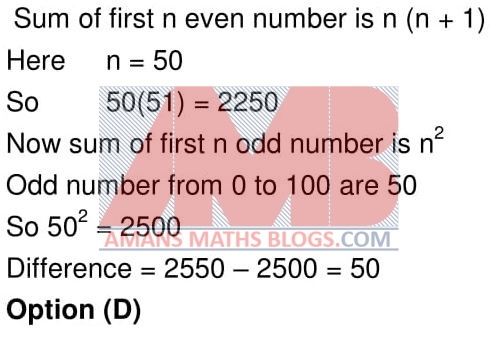NMTC 2017 Paper For Primary Level Ques No 4:

The value of (1/2 + 1/4 + 1/8)/(2 + 4 + 8) is

Options:

A. 16

B. 4

C. 1/4

D. 1/16

Solution: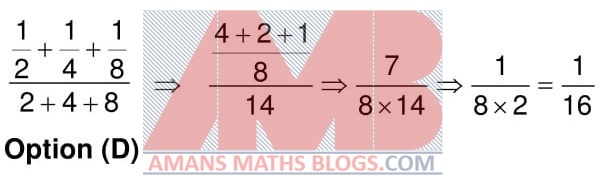NMTC 2017 Paper For Primary Level Ques No 5:

ABCD is a rectangle. AB = 8 cm and BC = 6 cm. Q is the midpoint of AB. P,R are on AD and BC respectively such that AP = 2 cm, CR = 1. Area of the shaded triangle in square cms is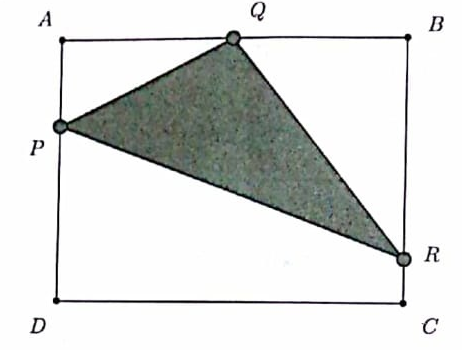Options:

A. 12

B. 13

C. 14

D. 16

Solution: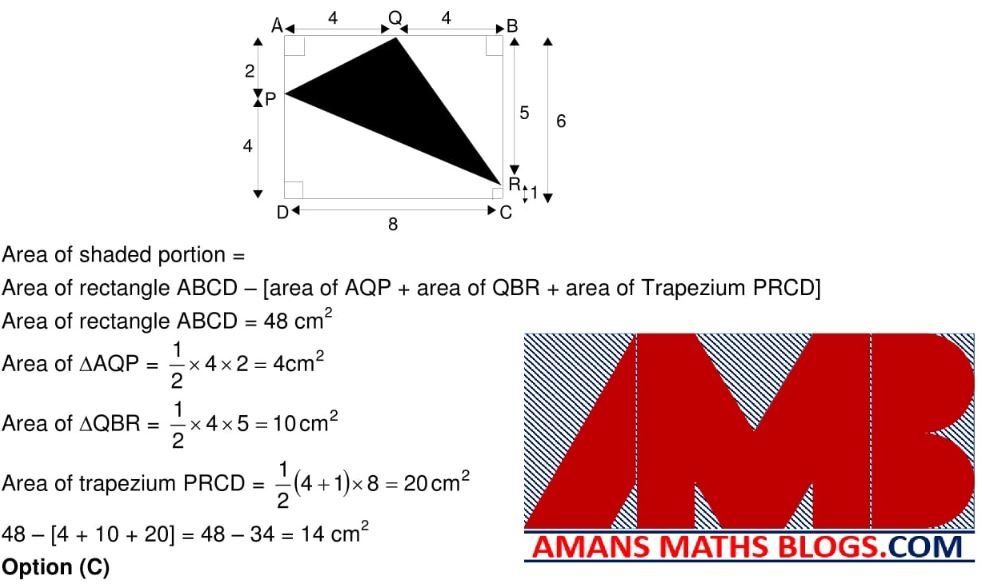NMTC 2017 Paper For Primary Level Ques No 6:

The Rishimoolam of a number is defined as follows. Consider the number 234. By multiplying its digits 2, 3 and 4, we obtain 2 x 3 x 4 = 24. Again, multiplying the digits of 24, we get 2 x 4 = 8. We say 8 is the Rishimoolam of the number 234. If 0 is the Rishimoolam, we say the number has no Rishimoolam. Which one of the following has no Rishimoolam?

Options:

A. 736

B. 647

C. 831

D. 619

Solution: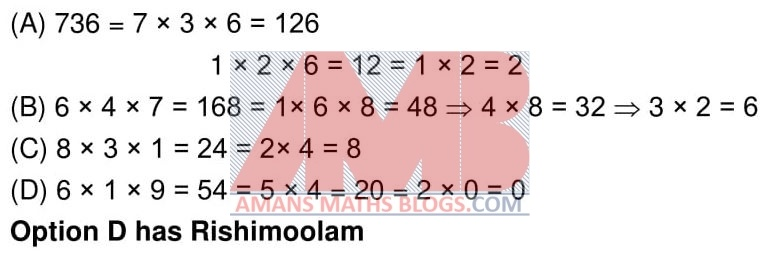NMTC 2017 Paper For Primary Level Ques No 7:

Two circles touch two parallel lines as shown in the diagram. The radius of each circle is 1 cm. The distance between the centers of the circle is 5 cm. The area of the shaded region is square cms is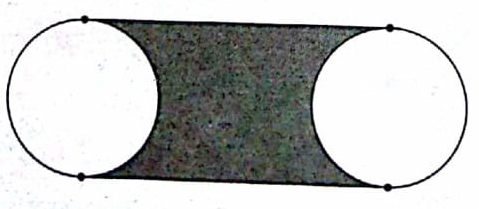Options:

A. 5Pi

B. 10Pi

C. 10 – Pi

D. 10 + Pi

Solution: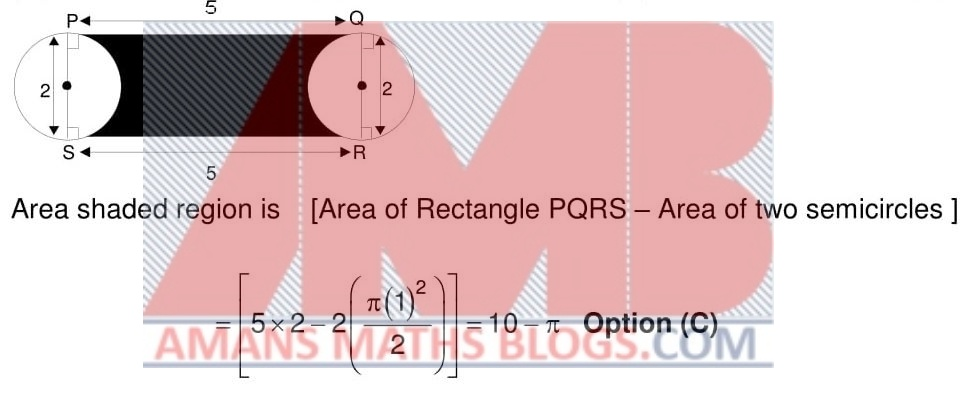NMTC 2017 Paper For Primary Level Ques No 8:

Samrud wrote two consecutive integers, one of which ends in a 5. He multiplied both. He squared the answer. The last two digits of his answer is

Options:

A. 50

B. 40

C. 10

D. 00

Solution:NMTC 2017 Paper For Primary Level Ques No 9:

Vishwa wrote a number on each side of 3 cards. In each cards, the numbers written on the sides are different. One side of each cards is a prime number and the other sides had 44, 59 and 38 respectively. Given that the sum of the numbers on each card is the same, the different between the largest and the second largest of the prime numbers on the cards is

Options:

A. 6

B. 7

C. 9

D. 4

Solution: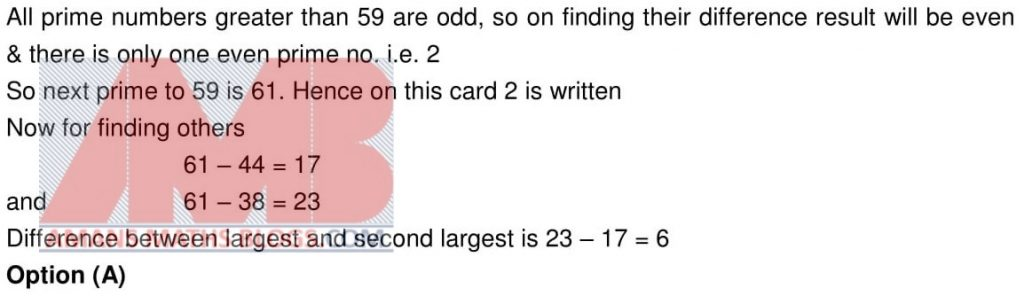NMTC 2017 Paper For Primary Level Ques No 10:

The number of three digit numbers abc such that a x b x c = 15 is

Options:

A. 2

B. 6

C. 8

D. 9

Solution:Part B: Instruction:

Write the correct answer in the space provided in the response sheet. For each correct response, you get 1 mark. For each incorrect response, you lose 1/4 mark.

NMTC 2017 Paper For Primary Level Ques No 11:

Five chairs cost as much as 12 desks, 7 desks cost as much as 2 tables and tables cost as much as 2 sofas. If the cost of 5 sofas is Rs. 5250, then the cost of a chair is Rs is_______.

Solution:NMTC 2017 Paper For Primary Level Ques No 12:

The average age of a class of 20 children is 12.6 years. 5 new children joined with an average age of 12.2 years. The new average of the class (to one decimal place) _______

Solution:NMTC 2017 Paper For Primary Level Ques No 13:

13 is a two digit prime and when we reverse its digits, the number 31 obtained is also a prime number. The number of two digit numbers having this property is______

Solution:NMTC 2017 Paper For Primary Level Ques No 14:

In a garden there are two plants. One plants is 44 cm tall and the other is 80 cm tall. The first plant grows 3 cm in every 2 months and the second 5 cm in every 6 months. The number of months after which the two plants will have equal height is______

Solution:NMTC 2017 Paper For Primary Level Ques No 15:

In 5 days a man walked a total of 85 KM. Every day he walked 4 KM less the previous day. The number of KM he walked on the last day is______

Solution:NMTC 2017 Paper For Primary Level Ques No 16:

In the adjoining figure, AB is parallel to CD. The value of x is______Solution:NMTC 2017 Paper For Primary Level Ques No 17:

In Mahadevans cycle shop for children, there are unicycles, having only one wheel, bicycles, having two wheels and tricycles, having three wheels. Samrud counts the seats and wheels and finds that there are totally 7 seats and 13 wheels. The number of bicycles is more than tricycles. The number of unicycles in the shop is______.

Solution:NMTC 2017 Paper For Primary Level Ques No 18:

There is a tree with several branches. Many parrots came to rest on the tree. When 6 parrots sat on each branch of the tree, all the branches were occupied but three parrots were left over. When 9 parrots sat on each branch, all parrots were seated but two branches were empty. If b is the number of branches and p is the number of parrots, the value of b + p is_______

Solution: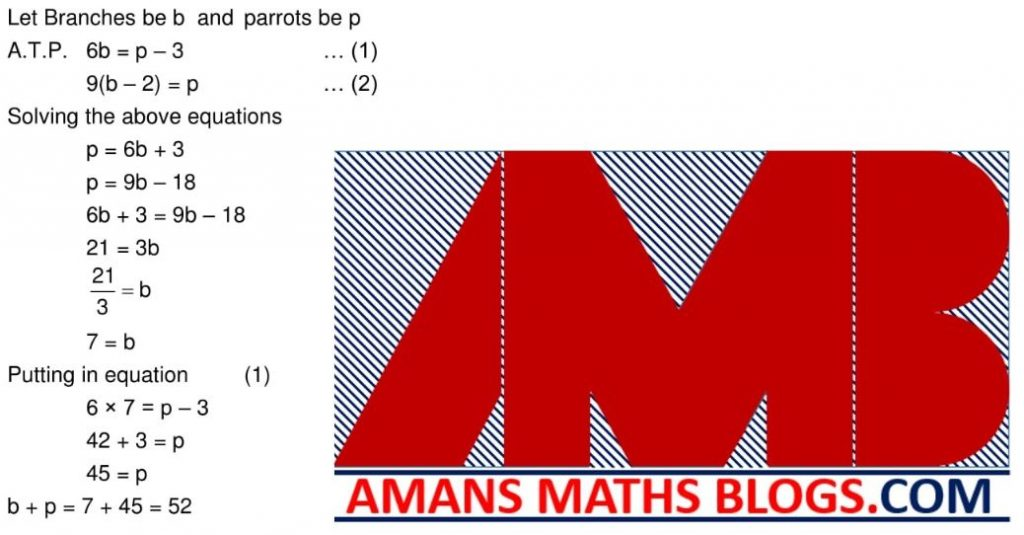NMTC 2017 Paper For Primary Level Ques No 19:

The incomes of A and B are in the ratio 3 : 2. Their expenditure are in the ratio 5 : 3. If each saves Rs 10,000, then As income is (in Rs.) _____.

Solution: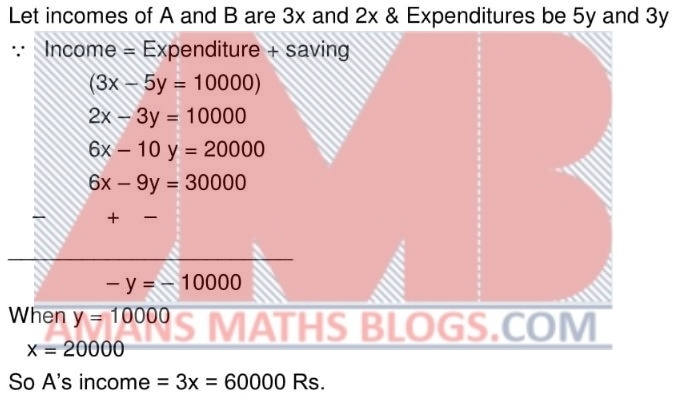NMTC 2017 Paper For Primary Level Ques No 20:

The radius of a circle is increased so that its circumference in increased by 5%. The area of the circle will increase by ______%.

Solution:

To get NMTC 2004 to 2018 Questions with Solutions, Click Image Below.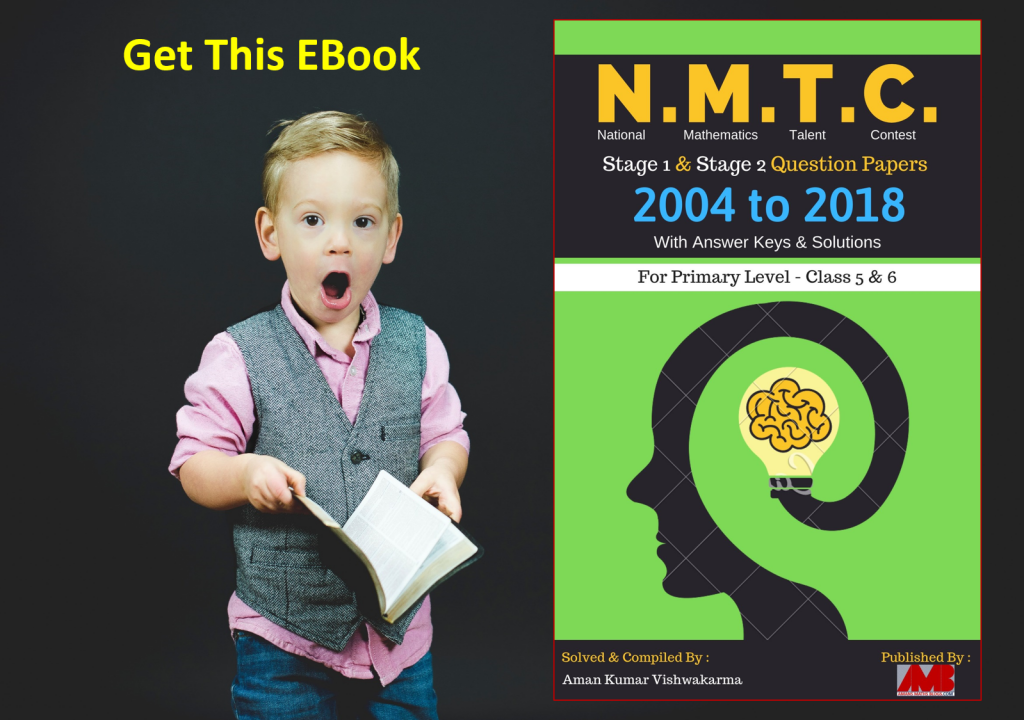Get Online Maths Tuition by AMBiPi for Class 8 to 12